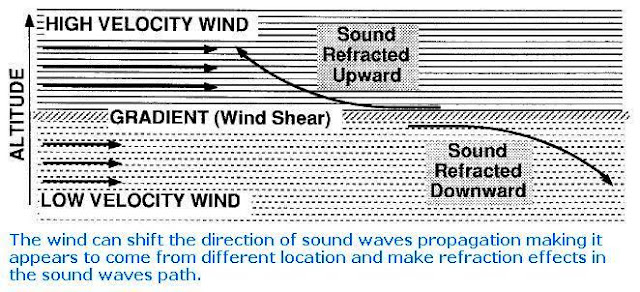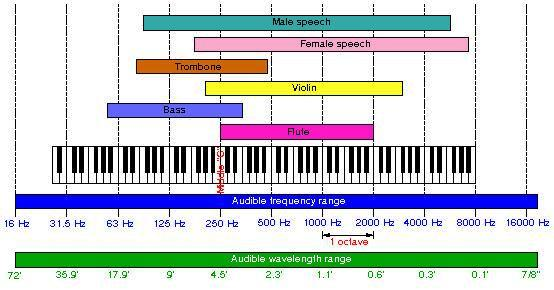### Properties of Sound

In the previous Topic, Fundamentals of Acoustics and Sound Systems, I listed the properties of sound which include:
1. Sound Propagation.
2. Wavelength and Cycle.
3. Amplitude and Loudness.
4. Frequency and Pitch.
5. Sound Velocity.
6. Complex Sound waves.
7. Octaves.
8. Timbre.

And today, I will explain these properties for more understanding of the Acoustics field.

1- Sound Propagation

Sound travels radially in all directions from the source, as shown in the fig.1Fig (1): Sound Propagation

The behavior of sound propagation is generally affected by three things:

• A relationship between density and pressure. This relationship, affected by temperature, determines the speed of sound within the medium (see fig.2)Fig (2): Temperature effect on Sound Waves

• The propagation is also affected by the motion of the medium itself. For example, sound moving through wind. Independent of the motion of sound through the medium, if the medium is moving, the sound is further transported (see fig.3).Fig (3): Wind effect on Sound Waves
• The viscosity of the medium also affects the motion of sound waves. It determines the rate at which sound is attenuated. For many media, such as air or water, attenuation due to viscosity is negligible

2- Wavelength and Cycle

Cycle: is a single complete vibration which includes one Crest and one Trough.

Crest: The section of the wave that rises above the undisturbed position.

Trough: the section which lies below the undisturbed position.

Wavelength: The wavelength of a Sound wave is the horizontal distance between any two adjacent corresponding locations on the wave train (see fig.4), This distance is usually measured in one of three ways: crest to next crest, trough to next trough, or from the start of a wave cycle to the next starting point.

Wavelength is denoted by “λ” (Greek letter lambda).fig (4): Wavelength

Period : A period (represented by the symbol T) is the amount of time required to complete one full cycle (see fig.5), The period of a sound wave can be mathematically related to several other aspects of wave motion, including wave speed, frequency, and wavelength.fig (5): Period and Amplitude

3- Amplitude and Loudness

Amplitude:

The amplitude of a sound is represented by the height of the wave. When there is a loud sound, the wave is high and the amplitude is large (see fig.5), Conversely, a smaller amplitude represents a softer sound. It represents the intensity of the sound corresponding to the loudness (volume).

The energy of a wave is proportional to the square of its amplitude. Therefore the intensity of a wave is also proportional to the square of its amplitude.

4- Frequency and Pitch

Frequency: It is calculated as the number of cycles per unit of time. Frequency is denoted by “f”, Units of hertz (Hz).

Note: The range of human hearing is approximately 20 Hz to 20 kHz (see fig.6)fig (6): Audible Sound Frequency Range

Pitch: It is an ears’ response to sound wave frequencies, it is closely related to frequency, but the two are not equivalent. Pitches are usually quantified as frequencies in cycles per second, or hertz, Low frequency (pitch) is characteristic of bass, an example of high frequency (pitch) is hissing.

5- Sound Velocity

It is the distance travelled during a unit of time by a sound wave propagating through an elastic medium.

Sound travels much faster in denser mediums than less dense mediums. For this reason, sound velocity is very slow (relatively) in air. Typical sound velocities are shown in the table below:

 Sound Velocity through various mediums Medium Sound Velocity (ft/sec) Air (70 F) 1,100 Water 4,500 Concrete 10,200 Steel 17,100

In the next Topic, I will continue explaining other Properties of Sound. Please keep following.